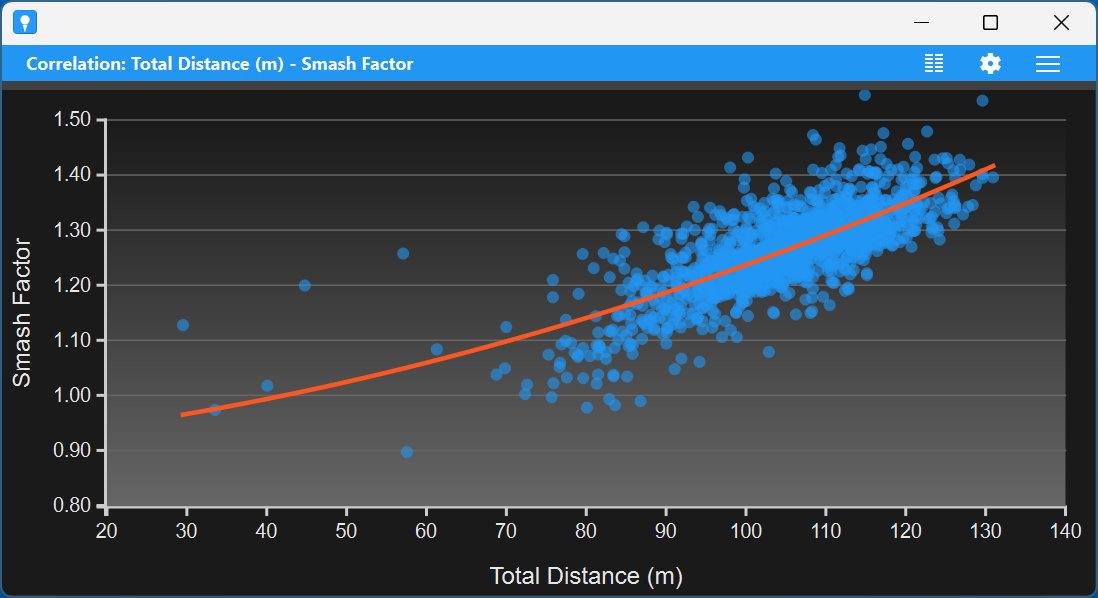## Correlation chartThe correlations chart component is meant to inspect the relation between two metrics. For example, you can use it to see how the total distance depends on the smash factor or on the club head speed.

The chart shows the relation between two metrics. One is plotted along the horizonal axis and the other along the vertical axis. Each point represents a shot with that combination of the two values for the metrics. If there is a positive relation between the metrics you expect the points to go from the lower left corner to the upper right corner. For example you expect this to be the case for the relation between the smash factor and the total distance. In other cases there is a negative relation. For example, it might be the case that the larger your club head speed, the smaller the smash factor becomes. And some relations will look more like a bell curve. For example, there will be an optimal launch angle to get the largest distance. You can use the charts to determine these.

If you hold the left mouse button while hovering over the chart you will get the exact metric values.

The chart has a menu in the right of the title bar. Here you have the following options:

• Select metrics. There is also an icon for this in the title bar. Here you can select the metrics you want to show in the chart. Select both the metrics in the lists and press OK.
• Save as PNG image. Save an image of the chart in a PNG file.
• Change settings. Change the settings. See below.
• Pop-out. Pop-out the chart as a separate window.

### Settings

The correlation chart component has a number of settings that you can change by clicking the settings icon in the title bar.

• General
• Name. The name of the component.
• Title. The title shown in the title bar. When you leave this empty, the metric names are shown in the title bar.
• Hor. metric. Select the metric that is used along the horizontal axis.
• Vert. metric. Select the metric that is used along the vertical axis.
• Use absolute value. Use the absolute values of the metrics. So the chart will e.g. use the absolute deviation distance or side spin.
• Remove outliers. Often there are some outliers in your data. Here you can indicate that a certain percentage of outliers is removed from the chart. Default this is 0%, so no outliers are removed. We use a rather simple algorithm to remove outliers. If you select e.g. 20%, 5% (with a minimum of 1) is removed in each direction. If you want a more involved method to remove outliers, you can define a filter for this in the data setting (see below).
• Fit curve. To get better insight in the correlation, you can fit a curve through the data points. You have the following possibilities:
• none. No curve is shown.
• regression line. This shows a line that best fits the data.
• regression curve. In this case a curve is fit through the data. This uses so-called quadratic polynomial regression.
• rolling average. This takes averages of 20% of the data values and shows how that changes over the horizontal axis. (This cannot be shown for the first and last 10% of the data.)
• Data. Here you can define a filter in the form of a formula. Only shots that satisfy the filter are taken into account in the chart.
• Appearance

Note that there are buttons to reset the settings to the default (as defined in the dashboard) and to copy the settings from another correlation chart component.